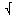# U+221A SQUARE ROOT

U+221A was added to Unicode in version 1.1 (1993). It belongs to the blockMathematical Operators in the Basic Multilingual Plane.

This character is a Math Symbol and is commonly used, that is, in no specific script. The character is also known as radical sign.

The glyph is not a composition. It has a Ambiguous East Asian Width. In bidirectional context it acts as Other Neutral and is mirrored. In text U+221A behaves as Ambiguous regarding line breaks. It has type Other for sentence and Other for word breaks. The Grapheme Cluster Break is Any.

The Wikipedia has the following information about this codepoint:

In mathematics, a square root of a number a is a number y such that y2 = a, in other words, a number y whose square (the result of multiplying the number by itself, or y × y) is a. For example, 4 and −4 are square roots of 16 because 42 = (−4)2 = 16.

Every non-negative real number a has a unique non-negative square root, called the principal square root, which is denoted by √a, where √ is called the radical sign or radix. For example, the principal square root of 9 is 3, denoted √9 = 3, because 32 = 3 × 3 = 9 and 3 is non-negative. The term whose root is being considered is known as the radicand. The radicand is the number or expression underneath the radical sign, in this example 9.

Every positive number a has two square roots: √a, which is positive, and −√a, which is negative. Together, these two roots are denoted ± √a (see ± shorthand). Although the principal square root of a positive number is only one of its two square roots, the designation "the square root" is often used to refer to the principal square root. For positive a, the principal square root can also be written in exponent notation, as a1/2.

Square roots of negative numbers can be discussed within the framework of complex numbers. More generally, square roots can be considered in any context in which a notion of "squaring" of some mathematical objects is defined (including algebras of matrices, endomorphism rings, etc.)

## Representations

System Representation
8730
UTF-8 E2 88 9A
UTF-16 22 1A
UTF-32 00 00 22 1A
URL-Quoted %E2%88%9A
HTML-Escape &#x221A;
Wrong windows-1252 Mojibake â
HTML-Escape &radic;
HTML-Escape &Sqrt;
digraph RT
alias radical sign
LaTeX \surd
Encoding: EUC-KR (hex bytes) A1 EE
Encoding: IBM866 (hex bytes) FB
Encoding: JIS0208 (hex bytes) A2 E5
Encoding: JIS0208 (hex bytes) AD F5
Encoding: KOI8-R (hex bytes) 96
Encoding: KOI8-U (hex bytes) 96
Encoding: MACINTOSH (hex bytes) C3
Encoding: X-MAC-CYRILLIC (hex bytes) C3

## Complete Record

Property Value
Age (age) 1.1
Unicode Name (na) SQUARE ROOT
Unicode 1 Name (na1)
Block (blk) Math_Operators
General Category (gc) Math Symbol
Script (sc) Common
Bidirectional Category (bc) Other Neutral
Combining Class (ccc) Not Reordered
Decomposition Type (dt) None
Decomposition Mapping (dm)Lowercase (Lower)
Simple Lowercase Mapping (slc)Lowercase Mapping (lc)Uppercase (Upper)
Simple Uppercase Mapping (suc)Uppercase Mapping (uc)Simple Titlecase Mapping (stc)Titlecase Mapping (tc)Case Folding (cf)ASCII Hex Digit (AHex)
Alphabetic (Alpha)
Bidi Control (Bidi_C)
Bidi Mirrored (Bidi_M)
Bidi Paired Bracket (bpb)Bidi Paired Bracket Type (bpt) None
Cased (Cased)
Composition Exclusion (CE)
Case Ignorable (CI)
Full Composition Exclusion (Comp_Ex)
Changes When Casefolded (CWCF)
Changes When Casemapped (CWCM)
Changes When NFKC Casefolded (CWKCF)
Changes When Lowercased (CWL)
Changes When Titlecased (CWT)
Changes When Uppercased (CWU)
Dash (Dash)
Deprecated (Dep)
Default Ignorable Code Point (DI)
Diacritic (Dia)
East Asian Width (ea) Ambiguous
Extender (Ext)
FC NFKC Closure (FC_NFKC)Grapheme Cluster Break (GCB) Any
Grapheme Base (Gr_Base)
Grapheme Extend (Gr_Ext)
Hex Digit (Hex)
Hangul Syllable Type (hst) Not Applicable
Hyphen (Hyphen)
ID Continue (IDC)
Ideographic (Ideo)
ID Start (IDS)
IDS Binary Operator (IDSB)
IDS Trinary Operator and (IDST)
InMC (InMC)
Indic Positional Category (InPC) NA
Indic Syllabic Category (InSC) Other
ISO 10646 Comment (isc)
Joining Group (jg) No_Joining_Group
Join Control (Join_C)
Jamo Short Name (JSN)
Joining Type (jt) Non Joining
Line Break (lb) Ambiguous
Logical Order Exception (LOE)
Math (Math)
Noncharacter Code Point (NChar)
NFC Quick Check (NFC_QC) Yes
NFD Quick Check (NFD_QC) Yes
NFKC Casefold (NFKC_CF)NFKC Quick Check (NFKC_QC) Yes
NFKD Quick Check (NFKD_QC) Yes
Numeric Type (nt) None
Numeric Value (nv) NaN
Other Alphabetic (OAlpha)
Other Default Ignorable Code Point (ODI)
Other Grapheme Extend (OGr_Ext)
Other ID Continue (OIDC)
Other ID Start (OIDS)
Other Lowercase (OLower)
Other Math (OMath)
Other Uppercase (OUpper)
Pattern Syntax (Pat_Syn)
Pattern White Space (Pat_WS)
Quotation Mark (QMark)
Radical (Radical)
Sentence Break (SB) Other
Simple Case Folding (scf)Script Extension (scx) Common
Soft Dotted (SD)
STerm (STerm)
Terminal Punctuation (Term)
Unified Ideograph (UIdeo)
Variation Selector (VS)
Word Break (WB) Other
White Space (WSpace)
XID Continue (XIDC)
XID Start (XIDS)
Expands On NFC (XO_NFC)
Expands On NFD (XO_NFD)
Expands On NFKC (XO_NFKC)
Expands On NFKD (XO_NFKD)